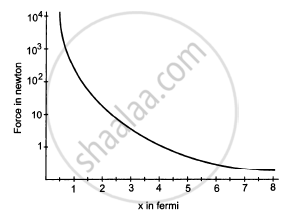Department of Pre-University Education, KarnatakaPUC Karnataka Science Class 11

# Suppose the Magnitude of Nuclear Force Between Two Protons Varies with the Distance Between Them as Shown in Figure. - Physics

Sum

Suppose the magnitude of Nuclear force between two protons varies with the distance between them as shown in figure. Estimate the ratio "Nuclear force/Coulomb force" for
(a) x = 8 fm
(b) x = 4 fm
(c) x = 2 fm
(d) x = 1 fm (1 fm = 10 −15m).#### Solution

First let us calculate the coulomb force between 2 protons for distance = 8 fm$F = \frac{K q^2}{r^2}$
$= \frac{9 \times {10}^9 \times (1 . 6 \times {10}^{- 19} )^2}{(8 \times {10}^{- 15} )^2}$
$= 3 . 6 N$
$F_N = 0 . 05 N$
$\frac{F_N}{F_C} = \frac{0 . 05}{3 . 6} = 0 . 0138 N$
For x= 4 fm
$F_C = \frac{9 \times {10}^9 \times (1 . 6 \times {10}^{- 19} )^2}{(4 \times {10}^{- 15} )^2}$
$= \frac{23 . 04 \times {10}^{- 29}}{(4 \times {10}^{- 15} )^2}$
$= 14 . 4 N$
$F_N = 1N$
$\frac{F_N}{F_C} = \frac{1}{14 . 4} = 0 . 0694 N$
$\text{ For }\ x = 2 \text{ fm }$
$F_C = \frac{9 \times {10}^9 \times (1 . 6 \times {10}^{- 19} )^2}{(2 \times {10}^{- 15} )^2}$
$= 57 . 6 N$
$F_N = 10 N$
$\frac{F_N}{F_C} = \frac{10}{57 . 6} = 0 . 173$
$\text{ For }\ x = 1 \text{ fm }$
$F_C = \frac{9 \times {10}^9 \times (1 . 6 \times {10}^{- 19} )^2}{(1 \times {10}^{- 15} )^2}$
$= 230 . 4 N$
$F_N = 1000 N$
$\frac{F_N}{F_C} = \frac{1000}{230 . 4} = 4 . 34$

Concept: Work Done by a Constant Force and a Variable Force
Is there an error in this question or solution?

#### APPEARS IN

HC Verma Class 11, Class 12 Concepts of Physics Vol. 1
Chapter 4 The Forces
Short Answers | Q 6 | Page 61
Share